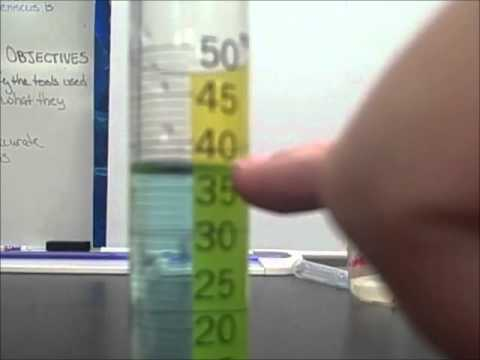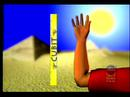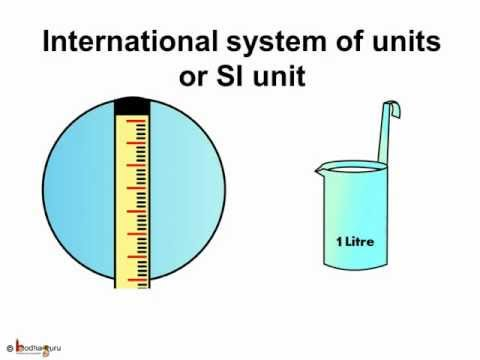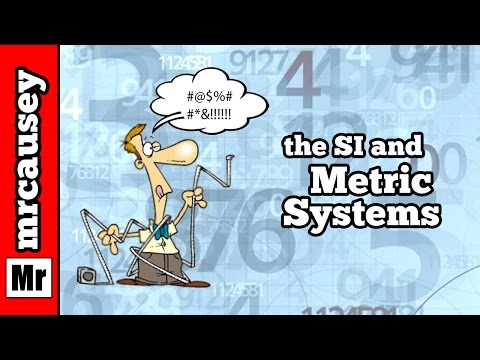## Measurement

Subject: Science

#### Overview

The process of comparing an unknown physical quantity with known standard quantity is called measurement. This note contains information about measurement and different quantities related to measurement.

##### Measurement

The accurate quantity of a substance can only be known by measurement. Measurement can be expressed in figures and in standard measurement. We use different instruments like measuring tape or scale for the measurement of length, beam balance for measuring masses and clock for the measurement of time.

The process of comparing an unknown physical quantity with a known standard quantity of the same kind is called measurement. Measurement is essential to know the exact physical quantity of the substances in our daily life. Those substances which are available in our surrounding can be measured are known as physical quantities. Some of the examples of physical quantities are the area, mass, time, length, temperature, etc. Those things which cannot be measured are called non- physical quantity. Some of the examples of non- physical quantity are love, kindness, anger, interest, feelings etc. There are two types of physical quantities. They are discussed below,

1. Fundamental Physical Quantity
2. Derived Physical Quantity

#### Fundamental quantity and derived quantity

1. Fundamental Physical Quantity: Those physical quantities, which can neither be derived from other quantities nor be further resolved into simpler ones, are called fundamental quantities. The units of fundamental quantities are called fundamental units or basic units. For example, the length of a body is the fundamental quantity as it cannot be expressed in terms of another quantity.
2. Derived Physical Quantity: Those physical quantities, which depend on two or more fundamental quantities or power of the fundamental quantity, are called derived quantities. The units of derived quantities are called derived units. For example, an area is a derived quantity. Since area= l × b, so the value of area depends on the value of length and breadth.

#### Unit

Without measurement, we cannot estimate the total amount of physical quantity.

Fundamental unit

Those units whose value do not depend on any other units are fundamental units. For example, Length of an object i.e meter, the mass of an object i.e kilogram, time i.e second.

Here are the seven fundamental units used to measure the fundamental quantities:

 Fundamental quantity Unit Symbol Length Meter m Mass Kilogram kg Time Second s Temperature Kelvin K Electric current Ampere A Luminous intensity Candela cd Amount of substance Mole mol

Derived Unit

Those units whose value depend on other units are derived units. For example, To measure the velocity of a car we must know the distance traveled by car and time taken by the car to travel the distance.

 S.N Derived Quantities Derived Units Symbol 1. Area Square meter m2 2. Volume Cubic meter m3 3. Density Kilogram per cubic meter kg/ m3 4. Speed/Velocity Meter per second m/s 5. Acceleration Meter per second per second m/s2 6. Force Newton N 7. (work/energy) Joule J 8. Power Watt W 9. Pressure Pascal Pa

#### Some information on system of units

There are different units of fundamental quantities that combine together to form a system of units. There are four systems of unit of measurement:
A. FPS system:

The full form of this system is Foot- Pound and second system. In this system, the fundamental units of length, mass and time are foot, pound and second.

B. CGS system

The full form of this system is Centimeter- Gram- Second system. In this system, the fundamental units of length, mass and time are centimetres, gram, and second.

C. MKS system

The full form of this system is Meter- Kilogram- Second system. In this system, the fundamental units of length, mass and time are meters, kilogram and second.

D. SI system

The full form of this system is System de international Unit. This system is modified form of MKS system. This system includes seven fundamental units such as the unit of electricity- Ampere (A), unit of temperature- kelvin (K), unit of light- Intensity (I) and the unit of amount of matter- mole (mol) along with three fundamental units of MKS system i.e. meter, kilogram and second.

##### Things to remember
• The process of comparing an unknown physical quantity with a known standard quantity is called measurement.
• Measurement is essential to know the exact physical quantity of the substances in our daily life.
• The units of length, mass and time are called fundamental units.
• System of Units: FPS system, CGS system, MKS system, SI system
• There are two types of physical quantities: fundamental and derived.
• It includes every relationship which established among the people.
• There can be more than one community in a society. Community smaller than society.
• It is a network of social relationships which cannot see or touched.
• common interests and common objectives are not necessary for society.
##### Videos for Measurement##### Measuring Volume Using Displacement##### Metric & Standard Measurement Systems##### Science - Measurement - What is measurement - English##### Questions and Answers
The process of comparing an unknown physical quantity with known standard quantity of the same kind is called measurement.

The substance which is available in our surroundings or can be measured is called a physical quantity. For example, length, mass, time, volume, etc.

The certain standard quantity which is used for the measurement of an unknown quantity is called unit of that quantity.

The fundamental unit is the unit which is independent of other units. In other words, the unit of length, mass and time are called fundamental units.

The physical quantity which is derived from other quantities and dependent on other physical quantities is called a derived unit.

A system of physical units based on the meter, kilogram, second and mole together with a set of prefixes to indicate multiplication or division by a power of ten is called SI unit.

Measurement is necessary for our daily life because the accurate quantity of a substance can be known through measurement.

 Fundamental unit Derived unit The unit of length, mass and time are the fundamental units. The unit made from fundamental units are derived unit. These quantities are directly measurable in a physical system. These quantities can be derived using fundamental units. Fundamental units are defined independently. These units are not defined independently.

Meter, Kilogram , Second , Kelvin , Ampere , Mole and Candela are units of SI system of measurement.

a) 300 cm

We have,

\begin{align*} 300 \: cm &= \frac {300}{100} \: m = 3\: m \:\:\: \left [ \because 1 \: m = 100 \: cm \right ] \\ \therefore 300 \: cm &= 3\: m \\ \end{align*}

b) 2.56 km

We have,

\begin{align*} 2.56 \: Km &= 2.56 \times 1000 \: m = 2560\: m \:\:\: \left [ \because 1 \: k m = 1000 \: m \right ] \\ \therefore 2.56\: Km &= 2560\: m \\ \end{align*}

c) 3.78 mm

We have,

\begin{align*} 3.78 \: mm = \frac {3.78}{10} \:c m = 0.387\: cm \:\:\: \left [ \because 1\: cm = 10 \: mm \right ] \\ \text {changing cm into mm} \\ 0.378 \: cm = \frac {0.378}{100} m = 0.378 \times 10^{-2} \:\:\: \left [ \because 1 \: m = 100 \: cm \right ] \\ \therefore 3.78 \: mm = 0.378 \times 10^{-2}\: m \\ \end{align*}